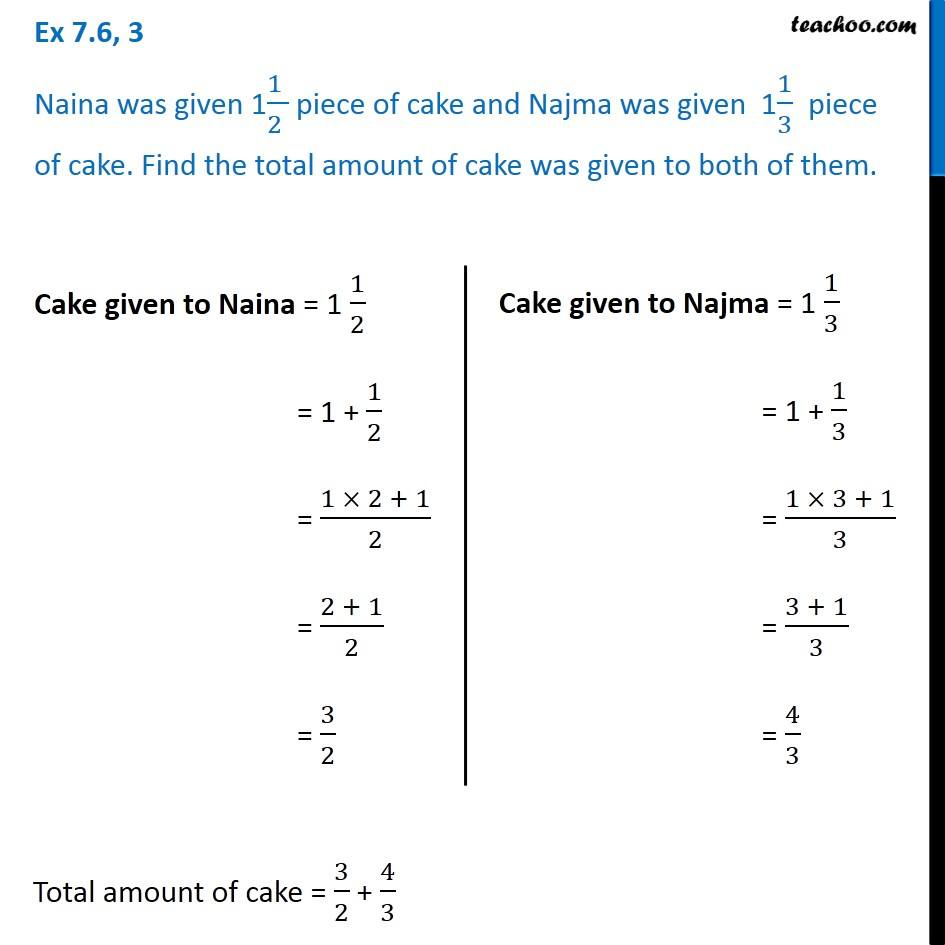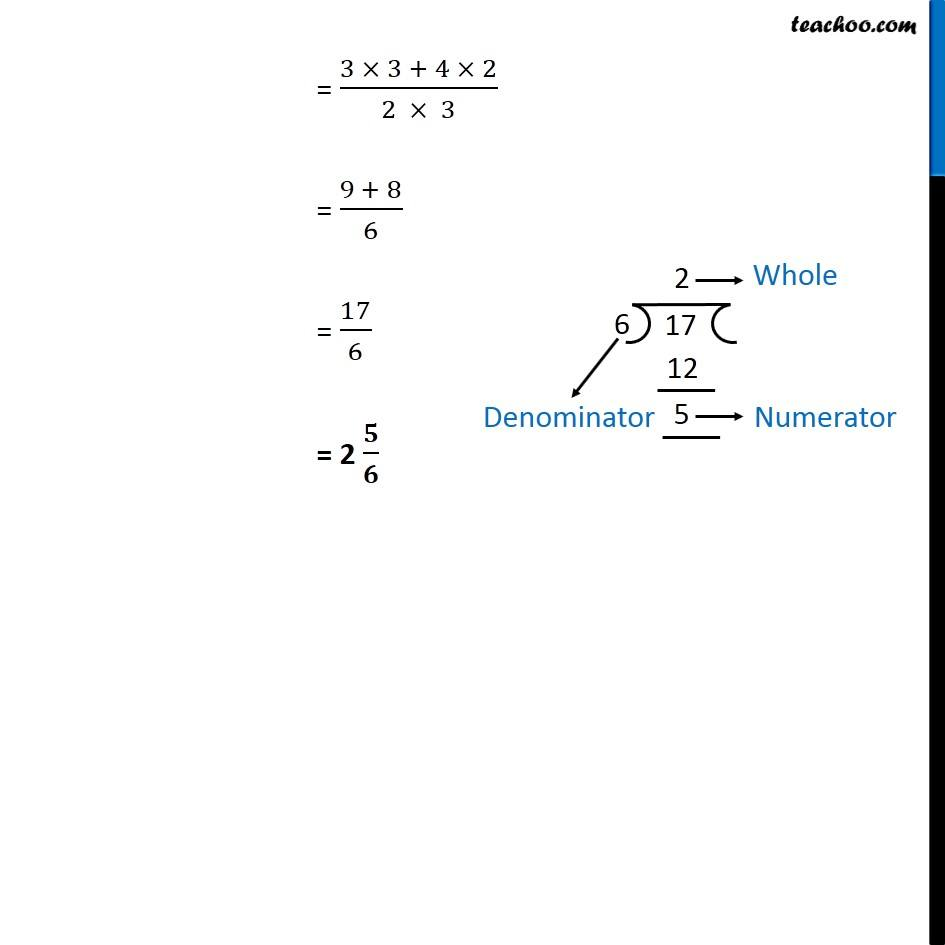Ex 7.6

Chapter 7 Class 6 Fractions
Serial order wiseLearn in your speed, with individual attention - Teachoo Maths 1-on-1 Class

### Transcript

Ex 7.6, 3 Naina was given 1(1 )/(2 ) piece of cake and Najma was given 11/3 piece of cake. Find the total amount of cake was given to both of them. Cake given to Naina = 1 1/2 = 1 + 1/2 = (1 × 2 + 1)/2 = (2 + 1)/2 = 3/2 Cake given to Najma = 1 1/3 = 1 + 1/3 = (1 × 3 + 1)/3 = (3 + 1)/3 = 4/3 Total amount of cake = 3/2 + 4/3 = (3 × 3 + 4 × 2)/(2 × 3) = (9 + 8)/6 = 17/6 = 2 𝟓/𝟔